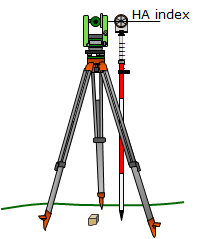### 2. Determining TSI elevation

#### a. TSI set up over known elevation

In this scenario the TSI is set up over a point whose elevation is known. The elevation must be transferred to the TSI by measuring the Height of the Instrument, HI, Figure F-3.Instrument Height Figure F-3

The TSI's elevation is calculated from:

 ElevTSI = Elev + HI Equation F-4 Elev: Point reference elevation

The HI is the vertical distance from the reference elevation to the TSI's Horizontal Axis (HA). Because the TSI is over the point, it is not possible to directly measure the HI. Most TSIs have an HA index mark on one or both standards which can be used to get a close value for the HI. There are two simple ways to measure the HI:

##### (1) Using a tape

A cloth tape or pocket-rod can be used to measure the distance from the elevation reference to the HA index mark, Figure F-4.Figure F-4 Measuring HI with Tape

##### (2) Using a prism pole

Another method is to place the prism pole next to the instrument and raise or lower the reflector so its center lines up with the HA index mark, Figure F-5. The prism pole reading is recorded as the HI.Figure F-5 Measuring HI with Prism Pole

Each method is subject to errors which will be discussed in a following section.

#### b. Sight to known elevation

The TSI is set up at a location from which a reference elevation or benchmark (BM) is visible. The vertical distance is measured to a reflector held on the BM, Figure F-6.Figure F-6 Using Benckmark to Establish TSI Elevation

The elevation of the TSI is determined from:

 ElevTSI = ElevBM + HRBM-VBM Equation F-5